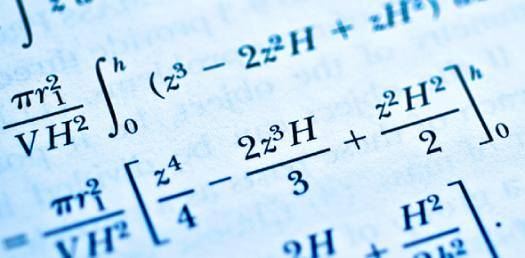# Statistics Chapter 2 Quiz

14 Questions | Total Attempts: 552Settings• 1.
The measures of central tendency measure which of the following:
• A.

The average or the middle

• B.

The spread of the data from the average

• C.

The difference between the highest and the lowest of the data

• D.

The number of data being used in an experiment

• 2.
The horizontal scale of a histogram is a great place to locate which of the following
• A.

Frequencies in the various classes

• B.

Identification of the population or sample of concern

• C.

Values for the class boundaries or class midpoints

• D.

All of the above

• 3.
The percentages in a pareto diagram are __________________________.
• 4.
A pareto diagram is popular in ___________________________________________ applications
• 5.
_______________________________ are values that divide the ranked data into quarters
• 6.
The first step in determining a percentile is to rank the data from highest to lowest.
• A.

True

• B.

False

• 7.
A normal histogram will be
• A.

Skewed to one side where one tail is stretched out longer than the other

• B.

Every value appears with equal frequency

• C.

There is no tail on the side of the class with the highest frequency

• D.

One side will mirror the other side

• 8.
The number of times an "x" value appears
• A.

Distribution

• B.

Frequency

• C.

Ogive

• D.

Mode

• 9.
A measure of position that allows you to see an overall "snapshot" of the data
• A.

Z-scores

• B.

Percentiles

• C.

Quartiles

• D.

5-number summary

• 10.
Kent scored a 92 on his test.  The mean score was an 87 with a standard deviation of 6.2.  What was the z-score for Kent's score.  **      Remember that z-scores are rounded to two decimal places
• 11.
The box and whiskers graph is a preferred way to display a 5-number summary
• A.

True

• B.

False

• 12.
Which of the following would be information contained in a five number summary?
• A.

The highest and lowest data value

• B.

The median

• C.

Quartile 1 and 3

• D.

First and third choices only

• E.

First, second, and third choices

• 13.
Briefly explain the steps for finding a quartile or a percentile.  Include the steps for when you obtain an integer or a decimal for the depth.
Related TopicsBack to top